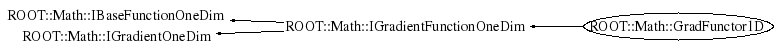```
Documentation for class Functor class.
It is used to wrap in a very simple and convenient way multi-dimensional function objects.
It can wrap all the following types:
<ul>
<li> any C++ callable object implemention double operator()( const double *  )
<li> a free C function of type double ()(double * )
<li> a member function with the correct signature like Foo::Eval(const double * ).
In this case one pass the object pointer and a pointer to the member function (&Foo::Eval)
</ul>
The function dimension is required when constructing the functor.

@ingroup  CppFunctions

```

## Function Members (Methods)

public:
private:
 virtual double DoDerivative(double x) const virtual double DoEval(double x) const

## Data Members

private:
 auto_ptr fImpl pointer to base gradient functor handler

## Class Charts## Function documentation

BaseFunc * Clone()
``` clone of the function handler (use copy-ctor)
```
double DoEval(double x) const
double DoDerivative(double x) const
```Default constructor

```
`{}`
```construct from an object with the right signature
implementing both operator() (double x) and Derivative(double x)

```
`{}`
```construct from a pointer to class and two pointers to member functions, one for
the function evaluation and the other for the derivative.
The member functions must take a double as argument and return a double

```
`{}`
```construct from two 1D function objects

```
`{}`
```Destructor (no operations)
`{}`